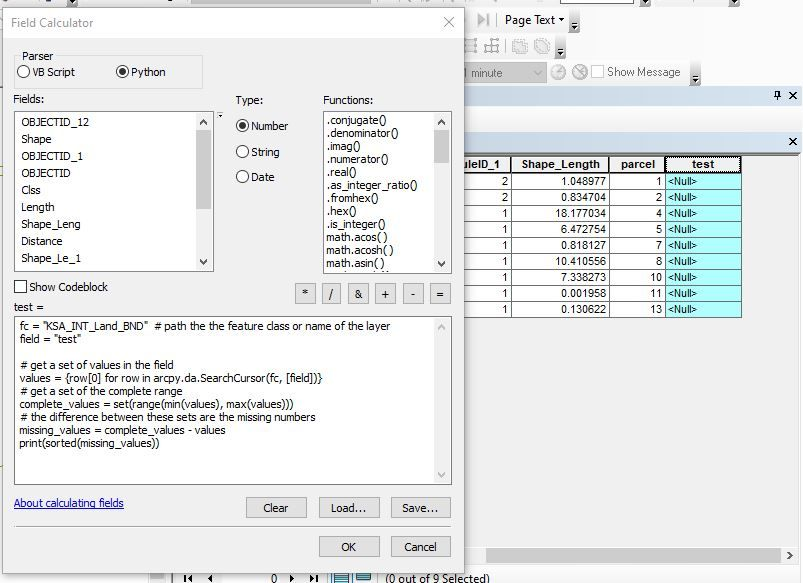# How Can I Find The Missing Sequence Number in a Field in ArcGIS

411
7
09-08-2023 12:20 AM
Labels (3)New Contributor III

How Can I Find The Missing Sequence Number in a Field in ArcGIS I have thousand numbers on that field ? I have ArcGIS 10.8Tags (3)
2 Solutions

Accepted SolutionsbyMVP Frequent Contributor

You can do it with a little Python script:

``````fc = "TestPolygons"  # path the the feature class or name of the layer
field = "IntegerField1"

# get a set of values in the field
values = {row for row in arcpy.da.SearchCursor(fc, [field])}
# get a set of the complete range
complete_values = set(range(min(values), max(values)))
# the difference between these sets are the missing numbers
missing_values = complete_values - values
print(sorted(missing_values))``````

Have a great day!
JohannesbyMVP Frequent Contributor
``````fc = "TestPolygons"  # path the the feature class or name of the layer
field = "IntegerField1"
group_field = "TextField1"

data = [row for row in arcpy.da.SearchCursor(fc, [group_field, field])]

# iterate over the groups
groups = {d for d in data}
for g in sorted(groups):
# get the values in that group
values = {d for d in data if d == g}
# get a set of the complete range
complete_values = set(range(min(values), max(values)))
# the difference between these sets are the missing numbers
missing_values = complete_values - values
print("Missing values in group {}: {}".format(g, sorted(missing_values)))``````

Have a great day!
Johannes
7 RepliesbyMVP Frequent Contributor

You can do it with a little Python script:

``````fc = "TestPolygons"  # path the the feature class or name of the layer
field = "IntegerField1"

# get a set of values in the field
values = {row for row in arcpy.da.SearchCursor(fc, [field])}
# get a set of the complete range
complete_values = set(range(min(values), max(values)))
# the difference between these sets are the missing numbers
missing_values = complete_values - values
print(sorted(missing_values))``````

Have a great day!
JohannesNew Contributor III

what should I change in your Python script to work , I create field test to run your Python script but it does not work ,  I want to know the missing number in field parcel ….I need more details!!!New Contributor IIIbyMVP Frequent Contributor

The script is meant to be run in the Python Window, not in the Fieled Calculator.

• Open the ArcMap Python Window
• Copy/Paste the script into the window
• Change the first two lines
• Execute by pressing Enter (may have to press it twice)

Have a great day!
JohannesNew Contributor III

Ok now its work …. but can we make another change in the python script to let him give us the missing number in group A and the missing number in group B , because the missing number in group A is different than the missing number in group B.byMVP Frequent Contributor
``````fc = "TestPolygons"  # path the the feature class or name of the layer
field = "IntegerField1"
group_field = "TextField1"

data = [row for row in arcpy.da.SearchCursor(fc, [group_field, field])]

# iterate over the groups
groups = {d for d in data}
for g in sorted(groups):
# get the values in that group
values = {d for d in data if d == g}
# get a set of the complete range
complete_values = set(range(min(values), max(values)))
# the difference between these sets are the missing numbers
missing_values = complete_values - values
print("Missing values in group {}: {}".format(g, sorted(missing_values)))``````

Have a great day!
JohannesNew Contributor III

Thank You Very Much For Your Great Solution